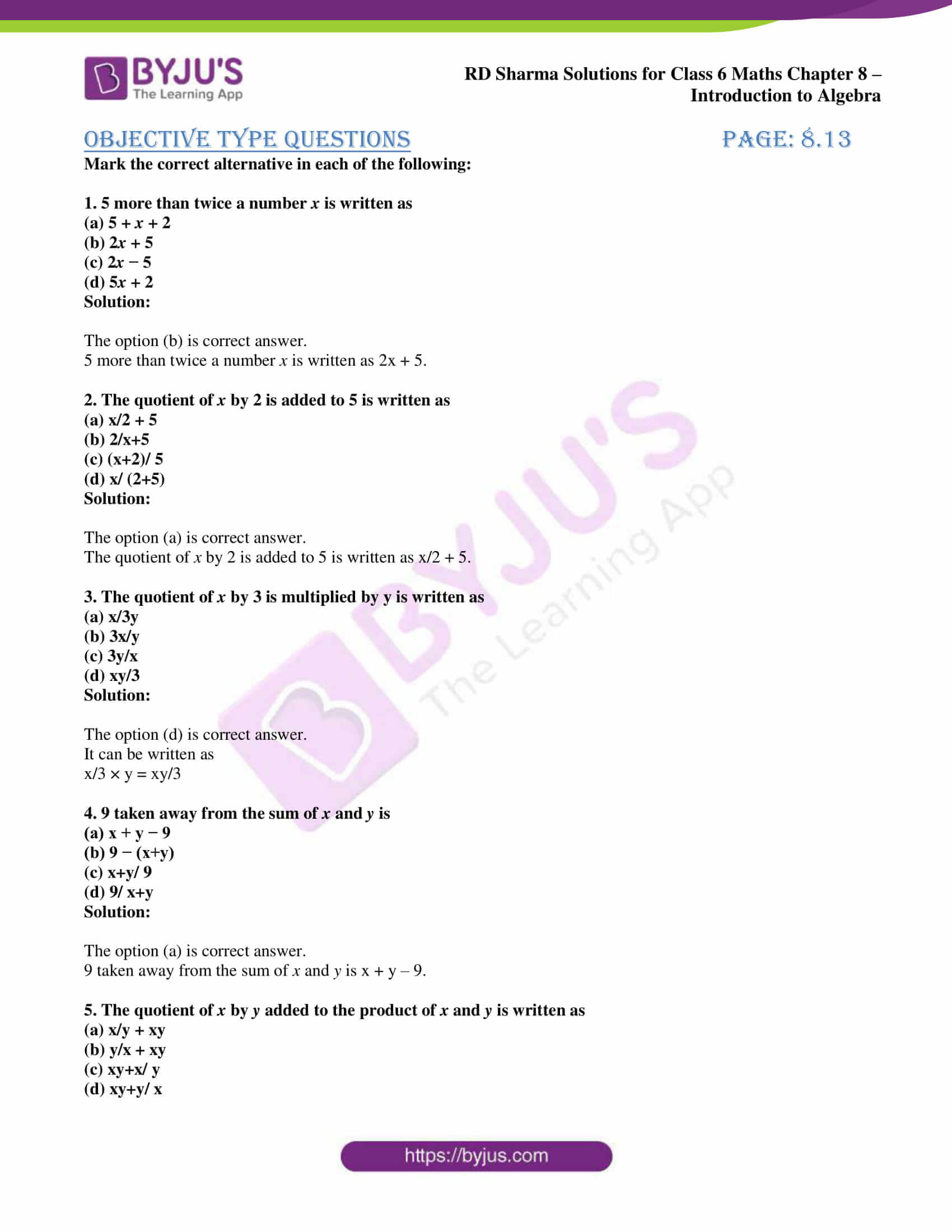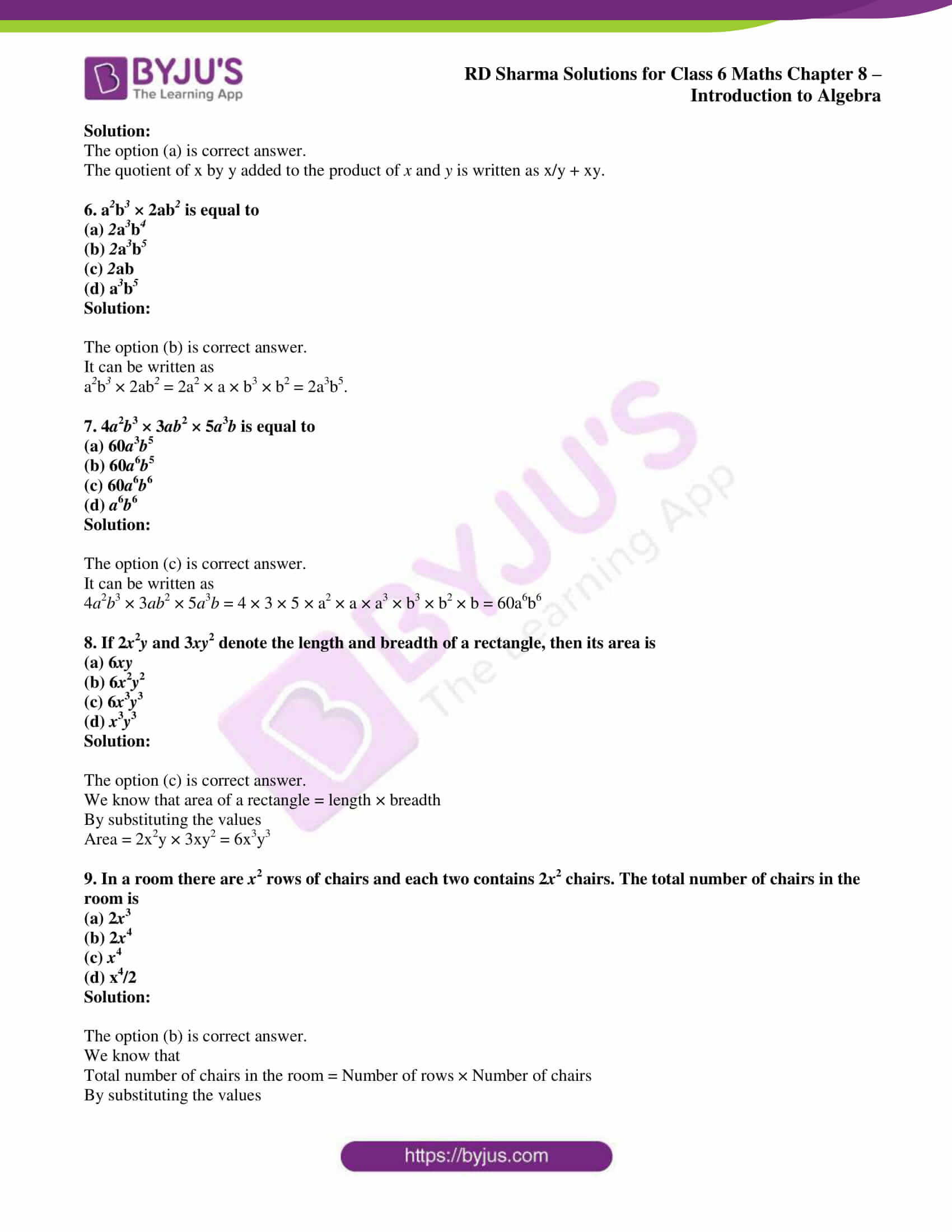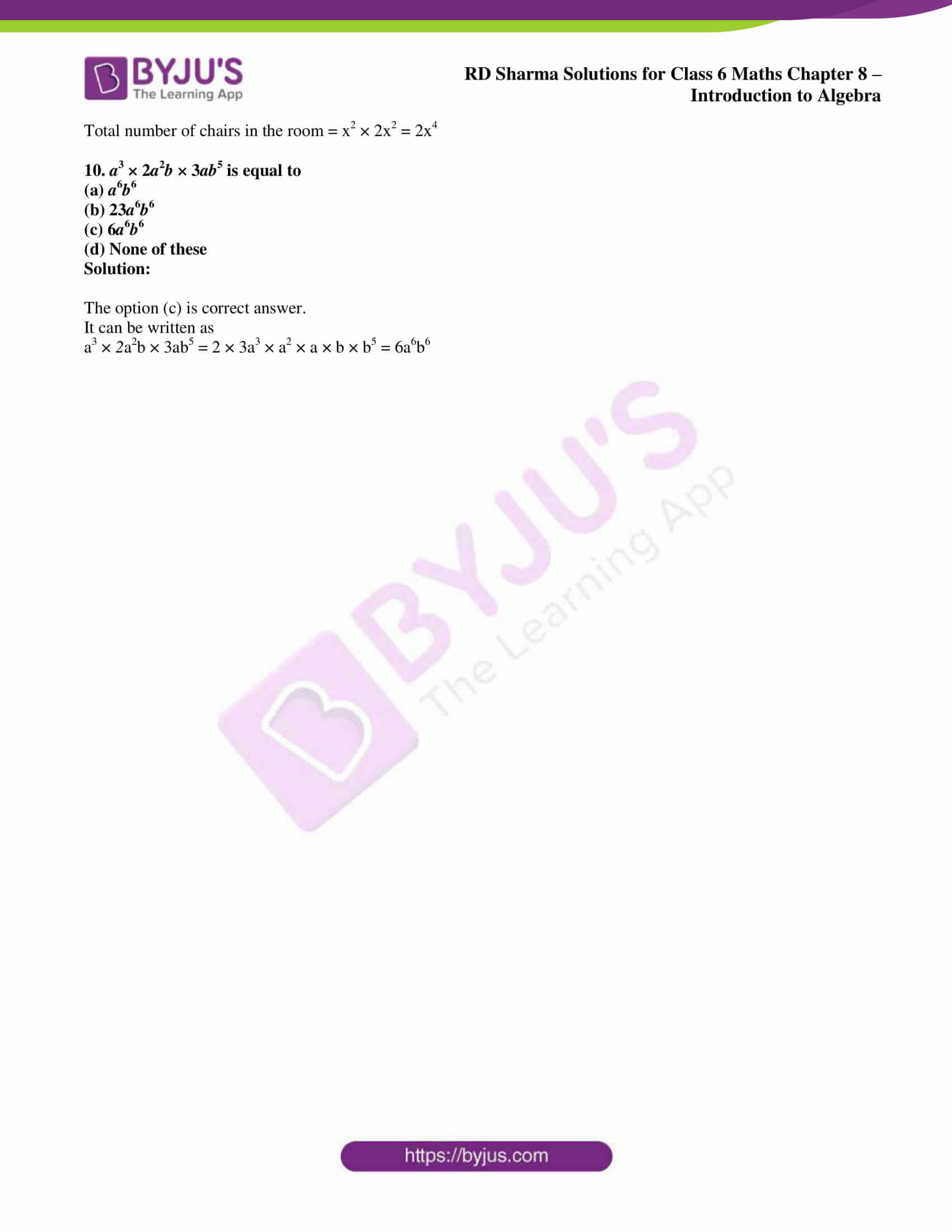# RD Sharma Solutions for Class 6 Maths Chapter 8: Introduction to Algebra Objective Type Questions

Until now, we were studying the arithmetic symbols known as numerals in the previous chapters. In this Chapter, the students mainly learn about the algebraic operations conducted on various equations. RD Sharma Solutions for Class 6 are well structured by faculty at BYJU’S after conducting vast research on the concepts. The primary objective is to help students improve their problem solving abilities to boost their exam preparation. RD Sharma Solutions for Class 6 Maths Chapter 8 Introduction to Algebra Objective Type Questions PDF’s are provided here.

## RD Sharma Solutions for Class 6 Maths Chapter 8: Introduction to Algebra Objective Type Questions Download PDF### Access answers to Maths RD Sharma Solutions for Class 6 Chapter 8: Introduction to Algebra Objective Type Questions

Mark the correct alternative in each of the following:

1. 5 more than twice a number x is written as
(a) 5 + x + 2
(b) 2x + 5
(c) 2x − 5
(d) 5x + 2

Solution:

The option (b) is correct answer.

5 more than twice a number x is written as 2x + 5.

2. The quotient of x by 2 is added to 5 is written as
(a) x/2 + 5
(b) 2/x+5
(c) (x+2)/ 5
(d) x/ (2+5)

Solution:

The option (a) is correct answer.

The quotient of x by 2 is added to 5 is written as x/2 + 5.

3. The quotient of x by 3 is multiplied by y is written as
(a) x/3y
(b) 3x/y
(c) 3y/x
(d) xy/3

Solution:

The option (d) is correct answer.

It can be written as

x/3 × y = xy/3

4. 9 taken away from the sum of x and y is
(a) x + y − 9
(b) 9 − (x+y)
(c) x+y/ 9
(d) 9/ x+y

Solution:

The option (a) is correct answer.

9 taken away from the sum of x and y is x + y – 9.

5. The quotient of x by y added to the product of x and y is written as
(a) x/y + xy
(b) y/x + xy
(c) xy+x/ y
(d) xy+y/ x

Solution:

The option (a) is correct answer.

The quotient of x by y added to the product of x and y is written as x/y + xy.

6. a2b3 × 2ab2 is equal to
(a) 2a3b4
(b) 2a3b5
(c) 2ab
(d) a3b5

Solution:

The option (b) is correct answer.

It can be written as

a2b3 × 2ab2 = 2a2 × a × b3 × b2 = 2a3b5.

7. 4a2b3 × 3ab2 × 5a3b is equal to
(a) 60a3b5
(b) 60a6b5
(c) 60a6b6
(d) a6b6

Solution:

The option (c) is correct answer.

It can be written as

4a2b3 × 3ab2 × 5a3b = 4 × 3 × 5 × a2 × a × a3 × b3 × b2 × b = 60a6b6

8. If 2x2y and 3xy2 denote the length and breadth of a rectangle, then its area is
(a) 6xy
(b) 6x2y2
(c) 6x3y3
(d) x3y3

Solution:

The option (c) is correct answer.

We know that area of a rectangle = length × breadth

By substituting the values

Area = 2x2y × 3xy2 = 6x3y3

9. In a room there are x2 rows of chairs and each two contains 2x2 chairs. The total number of chairs in the room is
(a) 2x3
(b) 2x4
(c) x4
(d) x4/2

Solution:

The option (b) is correct answer.

We know that

Total number of chairs in the room = Number of rows × Number of chairs

By substituting the values

Total number of chairs in the room = x2 × 2x2 = 2x4

10. a3 × 2a2b × 3ab5 is equal to
(a) a6b6
(b) 23a6b6
(c) 6a6b6
(d) None of these

Solution:

The option (c) is correct answer.

It can be written as

a3 × 2a2b × 3ab5 = 2 × 3a3 × a2 × a × b × b5 = 6a6b6

#### 1 Comment

1. HARSHINI

Interesting to me